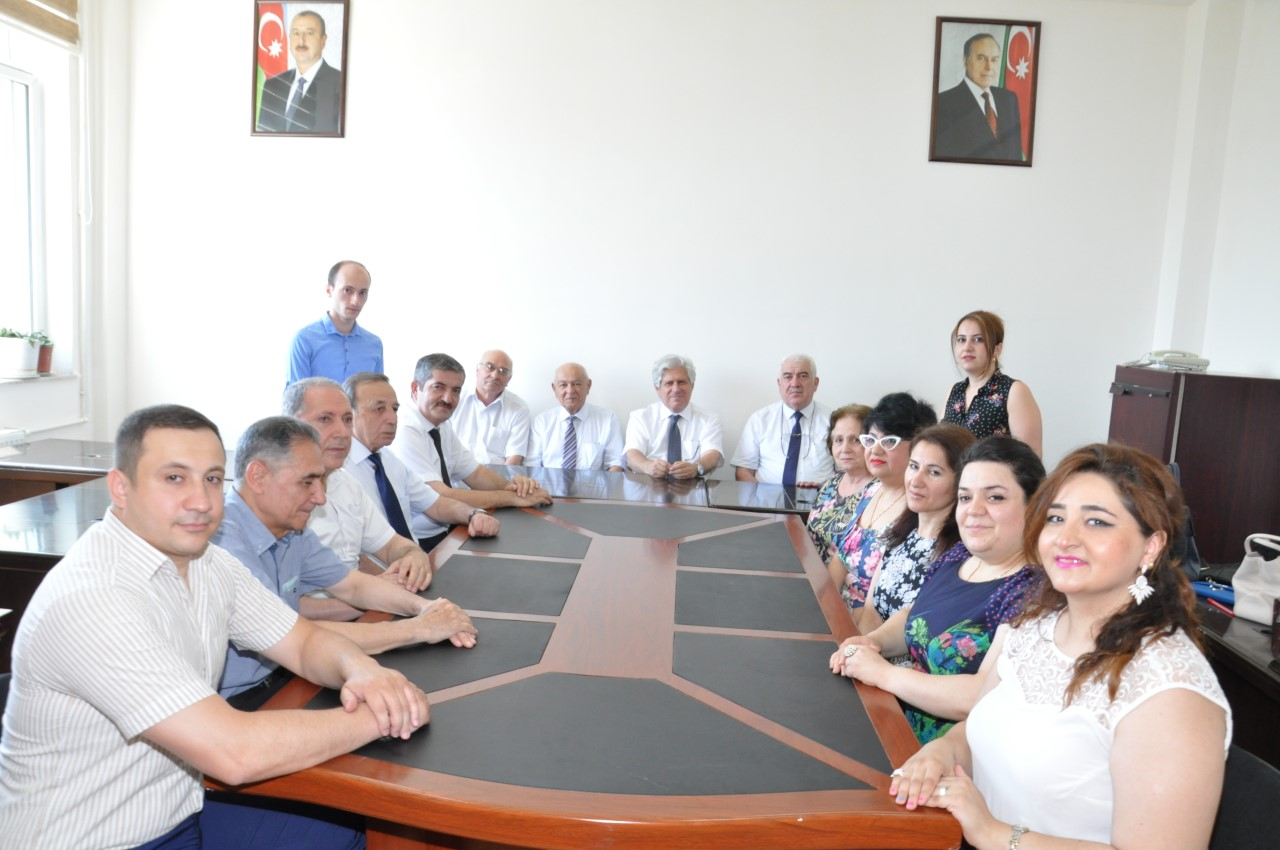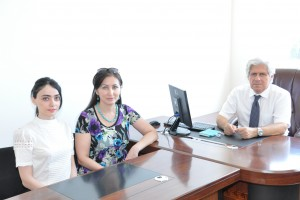﻿ BAKU STATE UNIVERSITY

## The chair of Algebra and geometryIn different years the department “Algebra and Geometry” has been operate under different names: “Geometry”, “Geometry and Algebra”, “Algebra and Topology”.

The chair “Geometry” was founded in Physico-mathematical faculty of Azerbaijan State University in 1935. In 1959 the chair obtained the name “Geometry and Algebra”. During the long time (1938-1972) the chief was the famous scientist and pedagog, corresponding member of ANAS, professor  M.A.Javadov. In 1972-2004 professor N.T. Abbasov was the chief of the chair “Geometry”.

In 1969 the chair “Geometry and Algebra” was divided into two parts: the chair “Geometry” and the chair “Algebra and Topology”.

Since 1969 to 1979 assoc.prof. M.R.Bunyatov was the chief of the chair “Algebra and Topology”, in 1980-1994 – assoc.prof. R.M.Sultanov and professor M.S.Akbarov were the chief of this chair. In 1994-2002 assoc.prof. V.A.Gasimov was the chief of the chair “Algebra and Topology”.

In 2002, “Geometry” and “Algebra and Topology” chairs were connected and has been operate under name “Geometry”. In 2004-2006  d.p.-m.s., professor  K.I.Khudaverdiyev was the chief of this chier.

In 2007  the chair “Geometry” was renamed as the chair “Algebra and Geometry”. In 2006-2017 assoc. prof. V.A.Gasimov was the chief of this chair.

Since 2017  d.p.-m.s., professor  A.A.Salimov is the chief of the chair “Algebra and Geometry”.

At present, the chair is functioning in the following content: (Head), c.p.-m.s., assoc. prof. A.F.Kocharli, c.p.-m.s., assoc. prof. N.Y.Aliyev, c.p.-m.s., assoc. prof. S.A.Bayramov, c.p.-m.s., assoc. prof. V.A.Gasimov (part time),  c.p.-m.s., assoc. prof. H.D.Fattayev,  c.p.-m.s., assoc. prof. H.M.Gasimov, c.p.-m.s., assoc. prof. O.M.Mammadov (part time), c.p.-m.s., assoc. prof. L.K.Hasanova, c.p.-m.s., assoc. prof.  A.A.Huseynova, c.p.-m.s., lecturer N.A.Sardarli (part time), senior lecturer L.M.Nasibova, lecturer S.F.Kazimova, lecturer G.M.Mammadova (part time), lecture S.T.Abdullayev (part time), senior laboratory assistant V.F.Guliyeva.

Primary areas of researchers of cheir are following:

Differential geometrical structures on manifold and geometries over algebras, Theory of lifts in tensor bundles, Boolean algebras and homologies, K-theory, C*- algebras, Hilbert modules, Lattice theory.

Salimov A.A. - Head, full professor, doctor of physical and mathematical sciences

Bayramov S.A. - professor, doctor of science in mathematics

Kocharli A.F. - associate professor, candidate of physical and mathematical sciences

Aliyev N.Y. - associate professor, candidate of physical and mathematical sciences

Gasimov V.A. - associate professor, candidate of physical and mathematical sciences

Fattayev H.D. - associate professor, candidate of physical and mathematical sciences

Gasimov H.M. - associate professor, candidate of physical and mathematical sciences

Mamedov O.M. - associate professor, candidate of physical and mathematical sciences

Hasanova L.K. - associate professor, candidate of physical and mathematical sciences

Huseynova A.A. - associate professor, candidate of physical and mathematical sciences

Sardarli N.A. - lecturer, candidate of physical and mathematical sciences

Nasibova L.M. - senior lecturer

Kazimova S.F. - lecturer

Abdullayev S.T. - lecturer

Guliyeva V.F. - senior laboratory assistant

On the step of baccalaureate following disciplines are taught:

1. Algebra and the theory of numbers

2. Contemporary problems of algebra

3. Algebra and analytical geometry

4. Analytical geometry

5. Analytical geometry and the highest algebra

6. Differential geometry and topology

7. Differential geometry and tensor calculus

8. Theory of the polynomials

On the step of "magistracy" in the specialty following disciplines are conducted:

1. Contemporary problems of science

2. History and the methodology of science

3. Geometry of imbedding manifolds

4. Non-Euclidean geometry

5. Spaces over algebras

6. Theory of lifts in tensor bundles

7. Lee groups and algebra Lee

8. Method of the exterior forms of Cartan

9. Algebraic topology, group theory

10. Theory of ideals

11. The Hilbert modules above S*-algebras

12. Universal algebra

And following disciplines are also conducted as selective objects:

1. Introduction into the theory of Morse

2. Some tasks of the moving frame in differential geometry

3. Some questions of the base of geometry

4. Elements of the theory of ideals

5. Commutative S*- algebras

6. Mathematical logic

7. Theory of the models